Spitzer Documentation & Tools
IRAC Instrument Handbook

## 4.4                  Color Correction

IRAC was a broad-band photometer. We provide here the prescription for how to interpret the data for sources with spectral shapes other than the nominal one assumed in the calibration process.

The conventions used by IRAC are the same as those used by IRAS (Beichman et al. 1988), COBE/DIRBE (Hauser et al. 1998), and ISO (Blommaert et al. 2003). The basic idea is to quote a flux density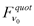at a nominal wavelength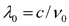that would be accurate for a source with a nominal spectrum,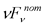=constant. Using this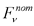is merely a matter of convention; in fact a wide range of spectra are expected, both redder (e.g., interstellar medium, asteroids) and bluer (e.g., stars) than nominal. The color correction tables given below allow observers to convert the nominally-calibrated data,, into more accurate estimates of the flux density at the nominal wavelength.

The number of electrons at a frequency ν  collected from the nominal source in a straight integration of duration t using a telescope with area A is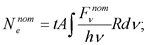(4.1)

where R is the system spectral response, h is the Planck constant, and ν is the frequency. The convention for R is that it is proportional to the number of electrons produced by a single photon with energy hν, where h is the Planck constant. If we define for convenience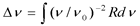, (4.2)

then the number of electrons collected from a source with the nominal spectrum is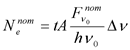(4.3)

The calibration factor, by which the number of electrons, Ne, from an arbitrary source must be divided in order to give the quoted flux density at the nominal wavelength, is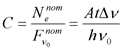(4.4)

The calibration factor is measured using observations of a celestial calibrator source of known spectrum,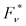. The number of electrons collected from the star is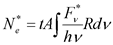(4.5)

Combining with equation (4.4), we can express the calibration factor in terms of the observed number of electrons from the calibration source:(4.6)

We can now cast this in convenient terms as follows: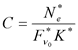, (4.7)

where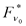is the flux density of the calibration source at the nominal wavelength, and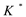is the color correction factor for the calibration source spectrum.

The color correction factor for a source with spectrum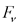is defined as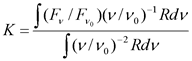. (4.8)

In this convention, the overall normalization of R is unimportant. Observers can correct the photometry to the spectrum of their source by either performing the integral in this equation, or looking up the color corrections for sources with similar spectra. Note that our definition of the color correction looks slightly different from that in the IRAS Explanatory Supplement, because we used the system spectral response R in electrons/photon, instead of ergs/photon.

We selected nominal wavelengths that minimize the need for color corrections, such that the quoted flux densities in IRAC data products are minimally sensitive to the true shape of the source spectrum. First, let us expand the source spectrum in a Taylor series about the nominal wavelength: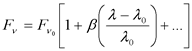. (4.9)

Using equation 4.8, the color correction for a source with spectrum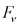is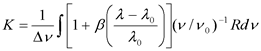(4.10)

The choice of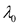that makes K minimally sensitive to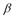is the one for which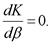Solving forwe get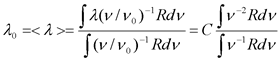(4.11)

So the optimum choice offor insensitivity to spectral slope is the weighted average wavelength.

Using the nominal wavelengths from

Table 4.3, the color corrections for a wide range of spectral shapes are less than 3%. Thus, when comparing IRAC fluxes to a theoretical model, placing the data points on the wavelength axis attakes care of most of the potential color dependence. To place the data points more accurately on the flux density axis (F* in Section 4.3), take the quoted flux densities (F*K* in Section 4.3 but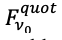here) derived from the images, and divide by the appropriate color correction factor in the tables below: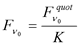. (4.12)

Or, calculate the color correction using equation 4.8 together with the spectral response tables, which are available in the IRAC section of IRSA’s Spitzer documentation website:

Table 4.3: IRAC nominal wavelengths and bandwidths. Rmax is the maximum spectral response.

 Channel λ0 (μm) Rmax Eff. Width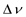(Eq. 4.2; 1012 Hz) Width/Rmax (1012 Hz) ˝-power wavelengths (μm)                               red                 blue 1 3.544 0.465 7.57 16.23 3.18 3.93 2 4.487 0.535 6.93 12.95 4.01 5.02 3 5.710 0170 1.93 11.70 5.02 6.44 4 7.841 0.318 3.94 12.23 6.44 9.37

Table 4.4 shows the color corrections for sources with power-law spectra,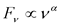, and Table 4.5 shows the color corrections for blackbody spectra with a range of temperatures. The nominal spectrum has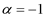, so the color corrections are unity by definition in that column. These calculations are accurate to » 1%. Note that the color corrections for a ν–1 and a ν0 spectrum are always unity. This is in fact a theorem that is easily proven using equations 4.8 and 4.11.

Table 4.4: Color corrections for power-law spectra,.

 Color correction for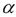= Channel -2 -1 0 1 2 1 1.0037 1 1 1.0037 1.0111 2 1.0040 1 1 1.0040 1.0121 3 1.0052 1 1 1.0052 1.0155 4 1.0111 1 1 1.0113 1.0337

Table 4.5: Color corrections for blackbody spectra.

 Temperature (K) Channel 5000 2000 1500 1000 800 600 400 200 1 1.0063 0.9990 0.9959 0.9933 0.9953 1.0068 1.0614 1.5138 2 1.0080 1.0015 0.9983 0.9938 0.9927 0.9961 1.0240 1.2929 3 1.0114 1.0048 1.0012 0.9952 0.9921 0.9907 1.0042 1.1717 4 1.0269 1.0163 1.0112 1.0001 0.9928 0.9839 0.9818 1.1215

Table 4.6 gives the color corrections for the spectrum of the zodiacal light, which is the dominant diffuse background in the IRAC wavelength range. The first model is the COBE/DIRBE zodiacal light model as implemented in Spitzer Planning Observations Tool Spot. The zodiacal light is mostly due to thermal emission from grains at ≈ 260 K over the IRAC wavelength range, except in channel 1, where scattering contributes ≈ 50% of the brightness. The second zodiacal light spectrum in Table 4.6 is the ISOCAM CVF spectrum (5.6 - 15.9 μm; Reach et al. 2003) spliced with the COBE/DIRBE model at shorter wavelengths. The two backgrounds differ because of different pointings.

Table 4.6: Color corrections for zodiacal light spectrum.

 COBE/DIRBE model ISOCAM+COBE/DIRBE Channel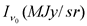K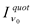K1 0.067 1.0355 0.069 0.40 1.0355 0.42 2 0.24 1.0835 0.26 1.44 1.0835 1.56 3 1.11 1.0518 1.16 6.64 1.0588 7.00 4 5.05 1.0135 5.12 25.9 1.0931 28.4

For sources with a complicated spectral shape the color corrections can be significantly different from unity. The corrections are infinite in the case of a spectrum dominated by narrow lines, because there may be no flux precisely at the nominal wavelength, which only demonstrates that such sources should be treated differently than continuum-dominated sources. We calculated one illustrative example which may prove useful. The ISO SWS spectrum of NGC 7023 is dominated by PAH emission bands and a faint continuum over the IRAC wavelength range. Table 4.7 shows the color corrections using equation 4.8 and the ISO spectrum. The large value in channel 1 is due to the presence of the 3.28 μm PAH band, which dominates the in-band flux relative to the weak continuum at the nominal wavelength of 3.550 μm. Channel 2 is mostly continuum. Channel 3 is dominated by a PAH band at 6.2 μm. Channel 4 has significant PAH band emission throughout, with prominent peaks at 7.7 and 8.6 μm. The values in this table can be used for comparison to IRAC colors of other sources by anti-color-correction, which gives the predicted colors for NGC 7023 in the same units as the SSC calibrated data: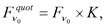which is shown in the last column of Table 4.7. Thus, PAH-dominated sources are expected to have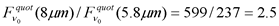(4.13)

Table 4.7: Color corrections for NGC 7023 (PAH-dominated) spectrum.

 Channel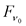K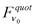1 17.3 2.21 38.3 2 30.3 1.21 36.6 3 169 1.40 237 4 1021 0.59 599

For observations of sources dominated by spectral lines, the quoted flux densities should be converted into fluxes using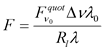, (4.14)

where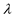is the wavelength of the spectral feature and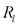is the spectral response at that wavelength. Both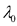and effective widthare in Table 4.3. The formalism used for continuum sources is inappropriate for spectral-line sources, because it is likely that both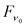and K can be infinity. It is important that the normalization of R used to determineandis the same. In Table 4.3, the column(effective width) was calculated with the same normalization of the response function as on the documentation website, so it is the appropriate one to use. The maximum response,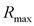is also given in that table, so the fluxes of lines near the center of the waveband can be estimated by simply multiplying the quoted flux densities by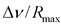, which is listed in the table in the column “Width.”